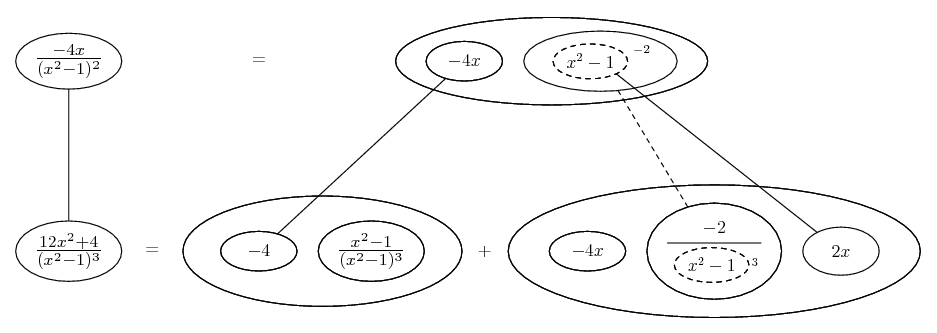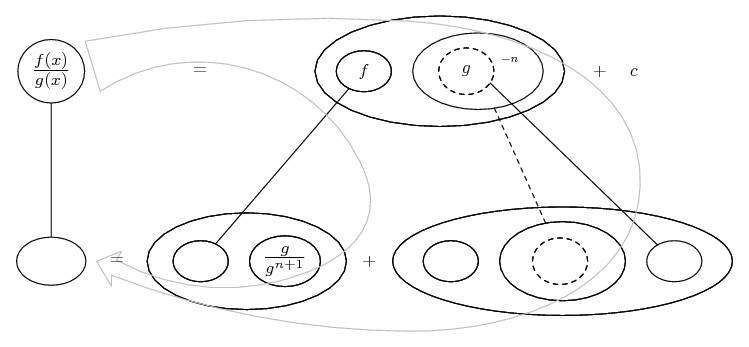# Thread: derivative - using quotient rule

1. ## derivative - using quotient rule

how do I get the derivative of:

-4x
---------
(x^2-1)^2

the answer is

12x^2+4
--------------
(x^2-1)^3

using the quotient rule, it seems simple enough but I cant seem to cancel out properly. Thanks!

2. Just in case a picture helps...... where... is the chain rule, wrapped inside...... the product rule. Straight continuous lines differentiate downwards (integrate up) with respect to x, and the straight dashed line similarly but with respect to the dashed balloon expression (the inner function of the composite which is subject to the chain rule).

The general drift is...Similar to the quotient rule, but no automatic squaring of the denominator.
_________________________________________

Don't integrate - balloontegrate!

Balloon Calculus; standard integrals, derivatives and methods

Balloon Calculus Drawing with LaTeX and Asymptote!

3.Originally Posted by calculus0how do I get the derivative of:

-4x
---------
(x^2-1)^2

the answer is

12x^2+4
--------------
(x^2-1)^3

using the quotient rule, it seems simple enough but I cant seem to cancel out properly. Thanks!
$\displaystyle y = \frac{-4x}{(x^2 - 1)^2}$

$\displaystyle \frac{dy}{dx} = \frac{(x^2 - 1)^2\,\frac{d}{dx}(-4x) - (-4x)\,\frac{d}{dx}[(x^2 - 1)^2]}{[(x^2 - 1)^2]^2}$

$\displaystyle = \frac{-4(x^2 - 1)^2 + 4x(2x)(2)(x^2 - 1)}{(x^2 - 1)^4}$

$\displaystyle = \frac{-4(x^2 - 1)^2 + 16x^2(x^2 - 1)}{(x^2 - 1)^4}$

$\displaystyle = \frac{(x^2 - 1)[-4(x^2 - 1) + 16x^2]}{(x^2 - 1)^4}$

$\displaystyle = \frac{-4x^2 + 4 + 16x^2}{(x^2 - 1)^3}$

$\displaystyle = \frac{12x^2 + 4}{(x^2 - 1)^3}$

$\displaystyle = \frac{4(3x^2 + 1)}{(x^2 - 1)^3}$.

4. Next time, show your efforts. Someone will be pleased to help you whereever you wander off.

5. Dear calculus0,

By the time I typed the answer it had already been given by other members. Hope these answers will help you.

6. THANKS! everyone.. that was so fast.

#### Search Tags

derivative, quotient, rule